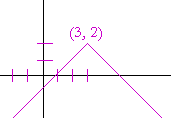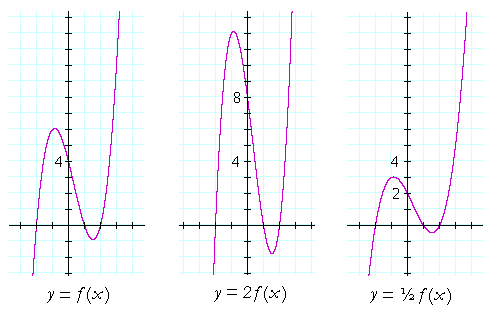Topics in

P R E C A L C U L U S

17

# TRANSLATIONS

The translation of a graph

A TRANSLATION OF A GRAPH is its rigid movement, vertically or horizontally.On the left is the graph of the absolute value function.  On the right is its translation to a "new origin" at (3, 4).

The equation of the absolute value function is

y = |x|.

The equation of its translation to (3, 4) is

y − 4 = |x − 3|.

We can see that when x = 3,  then  y − 4 = 0, that is, y = 4.

In general,

 If the graph of y = f(x) is translated a units horizontally and b units vertically, then the equation of the translated graph is y − b = f(x − a).

When f(x) is translated a units horizontally, then the argument of f(x) becomes xa.  In the example above, the argument of |x| becomes x − 3.

We will prove that below.

Example 1.   Write the equation of this graph:Answer.   y − 3 = |x + 5|.

The graph of the absolute value has been translated 3 units up, but 5 units to the left.  a = −5.  Therefore,  xa  becomes

x − (−5) = x + 5.

Problem 1.   Write the equation of this graph:(Hint: What graph was translated?)

The graph that was translated is

y = −|x|.

Problem 8 here.

It has been translated 4 units left and 3 units down. Its equation is

y + 3 = −|x + 4|.

Problem 2.   Sketch the graph of

y = |x − 3|.Problem 3.   Sketch the graph of

y = −|x + 2|.Problem 4.   Sketch the graph of

y = −|x − 3| + 2.

This is equivalent to  y − 2 = −|x − 3|.The graph is reflected about the x-axis, and then translated to (3, 2).

Problem 5.   Sketch the graph of  y =.This is the square root function translated 1 unit to the right.

Problem 6.   Sketch the graph of  y = −.This is the reflected square root function, translated 3 units to the left.

Problem 7.   Sketch the graph of  y = 1 − x2.This is equivalent to  y − 1 = −x2,  which is the reflected parabola translated 1 unit up.

Example 2.  The vertex of a parabola.   Write the equation of the parabola (with leading coefficient 1) whose vertex is at the point (a, b).Answer.    yb = (xa)2.   This is a translation of  y = x2  to (a, b).

Problem 8.   Write the equation of the parabola whose vertex is at

 a)  (1, 2) y − 2 = (x − 1)2 b)  (−1, 2) y − 2 = (x + 1)2 c)  (1, −2) y + 2 = (x − 1)2

Example 3.   What are the coördinates of the vertex of this parabola?

 y = x2 + 6x + 9 Solution.   To answer, we must make the equation look like this: y − b = (x − a)2

The vertex will then be at (a, b).

Now,  x2 + 6x + 9  is the perfect square of  (x + 3):

y = x2 + 6x + 9 = (x + 3)2.

Therefore, a = −3, and b = 0.  The vertex is at (−3, 0.)

Example 4.   What are the coördinates of the vertex of this parabola?

y = x2 + 5

Solution.   Again, we must make the equation look like this:

yb = (xa)2.

Upon transposing 5 --

y − 5 = x2

-- we see that a = 0, and b = 5.  The vertex is at (0, 5).

Example 5.  Completing the square.   What are the coördinates of the vertex of this parabola?

 y = x2 + 6x −2 Solution.   To make this form -- y − b = (x − a)2

-- we will transpose the constant term, and complete the square on the right.

 y + 2 = x2 + 6x Complete the square by adding 9 to both sides: y + 2 + 9 = x2 + 6x + 9 y + 11 = (x + 3)2

The vertex is at (−3, −11).

Problem 9.   What are the coördinates of the vertex of this parabola?

y = x2 − 10x + 25

The right-hand side is the perfect square of (x − 5).

y = (x − 5)2

The vertex therefore is at (5, 0).

Problem 10.   What are the coördinates of the vertex of this parabola?

y = x2 − 1

The equation implies

y + 1 = x2.

The vertex is at (0 −1).

Problem 11.   What are the coördinates of the vertex of this parabola?

y = x2 − 8x + 1

Transpose the constant term, and complete the square on the right:

 y − 1 = x2 − 8x y − 1 + 16 = x2 − 8x + 16 y + 15 = (x − 4)2

The vertex is at (4, −15).

The equation of a circle

The equation of any figure is the relationship between every coördinate pair on the figure. What, then, characterizes every point (x, y) on the circumference of a circle?Every point (x, y) is the same distance r from the center.  Therefore, according to the Pythagorean distance formula for the distance of a point from the origin:

x2 + y2 = r2.

That is the equation of a circle of radius r, with center at the origin (0, 0).

This, for example --

x2 + y2 = 25

-- is the equation of a circle of radius 5 centered at the origin.

Question.   What is the equation of a circle with center at (a, b) and radius r?

Answer.   (xa)2 + (yb)2 = r2.

The circle has been translated from (0, 0) to (a, b).

Problem 12.   Write the equation of the circle of radius 3, and center at the following point.

 a)   (1, 2) (x − 1)2 + (y − 2)2 = 9 b)   (−1, −2) (x + 1)2 + (y + 2)2 = 9 c)   (1, −2) (x − 1)2 + (y + 2)2 = 9

Example 6.   Show that the following is the equation of a circle.  Name the radius and the coördinates of the center.

x2 − 4x + y2 − 2y = 11

Solution.   To show that something is the equation of a circle, we must show that it can have this form:

(xa)2 + (yb)2 = r2.

Therefore, we will complete the square in both x and y.

To complete the square in x, we will add 4 to both sides.

To complete the square in y, we will add 1 to both sides.

 (x2 − 4x + 4) + (y2 − 2y + 1) = 11 + 4 + 1 (x − 2)2 + (y − 1)2 = 16.

This is the equation of a circle of radius 4, whose center is at (2, 1).

We can say, then, that when a quadratic in x plus a quadratic in y is equal to a number --

x2 − 4x  +  y2 − 2y = 11

-- then that is the equation of a circle.

The coefficients of x2 and y2 are 1. And the number must be greater than the negative of the sum of the squares of half the coefficients of x and y.

Problem 13.   Show that the following is the equation of a circle.  Name the radius and the coördinates of the center.

x2 + 6x + y2 + 10y − 2 = 0

Transpose the constant term, and complete the square in both x and y.  Add the same square numbers to both sides:

 (x2 + 6x + 9) + (y2 + 10y + 25) = 2 + 9 + 25 (x + 3)2 + (y + 5)2 = 36

This is the equation of a circle of radius 6, with center at (−3, −5).

Here is the proof of the main theorem.

Theorem.  If the graph of y = f (x) is translated a units horizontally and b units vertically, then the equation of the translated graph is

yb = f(xa).

For in a translation, every point on the graph moves in the same manner. Let (x1y1), then, be the coördinates of any point on the graph of y = f (x), so that

y1 = f (x1).

And let us translate the graph a units horizontally and b units vertically, so that x1 goes to the point

x1 + a,

and y1 goes to the point

y1 + b.

If a is a positive number, then that point will be to the right of x1, while if a is negative, it will be to the left.  Similarly, if b is a positive number, then y1 + b will be above y1, while if b is negative, it will be below.

Now, what will be the equation of the translated graph, such that when the value of x in the equation is x1 + a, the value of y will be y1 + b?

We say that the following is the equation:

yb = f(xa).

For, when x = x1 + a:

yb = f(x1 + aa) = f(x1) = y1;

y = y1 + b.

And (x1, y1) is any point on the graph of y = f (x).  Therefore the equation of the translated graph is

yb = f(xa).

Which is what we wanted to prove.

Vertical stretches and shrinks

If we multiply a function f(x) by a number c -- producing  c f(x) -- what will be the effect on the graph?If we multiply f(x) by a number greater than 1 -- as in the graph in the center -- then every y-value is stretched; in that graph, by a factor of 2.

But if we multiply f(x) by a number less than 1 -- as in the graph on the right -- then every y-value is shrunk; in that graph, by a factor of ½.Next Topic:  Rational functions

Please make a donation to keep TheMathPage online.
Even \$1 will help.